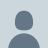## Time Conversion – How to convert 24hr input as 4-digit number into TIME in Excel?

How to convert something like 1200 into 12:00?For easy sake of input, we often input 24-hr time as four digit number like 930 for 9:30, 1815 for 18:15 etc.  However, if time is input in this way in Excel, you won’t get the advantage of performing time related calculation, e.g. time difference.

1815 – 900 = 885 instead of 8:45 as per require.

From data entry point of view, inputting time in time format by putting the colon in between hour and minute could be time consuming (comparing to just inputting four-digit number).

Luckily I found a solution in one of the posts answered by PaddyD in mrexcel forum (http://www.mrexcel.com/forum/excel-questions/752657-formula-involving-time-after-6-pm.html )to convert four-digit number into Time by a formula:

=ROUNDDOWN(INPUT,-2)/2400 + MOD(INPUT,100)/1440

where INPUT is a four-digit number, e.g. 0900, 1200, 1800 etc.

I found this formula is great and would like to share, with an explanation on how it works.Note: Remember to format the cells into the TIME format you need!

First of all, just to remind what TIME is in Excel.

Excel simply considers TIME as a value from 0 to 1, where 0 stands for 00:00; 1 stands for 24:00.  Every second is divided evenly.

Let’s put it into number for better illustration:

We have 24 hours in a day, thus 1 hour is interpreted as 1/24 = 0.041666667.

• 1:00am or 1 hour = 0.41666667
• 2:00am or 2 hours = 0.083333333
• 3:00am or 3 hours = 0.125
• etc…

Base on same ground, we have 60×24 =1440 minutes per day.  Each minute is interpreted as 1/1440 = 0.000694444444

Multiplying the above by 60 would give you exactly one hour or 0.41666667

With the understanding of this logic behind, the formula makes perfect sense.

=ROUNDDOWN(INPUT,-2)/2400+MOD(INPUT,100)/1440

The 1st part of the formula – Give you HOUR

ROUNDDOWN(INPUT,-2) gives a result from 0,100, 200, 300,… to 2400. How?

As the input is (supposedly) limited to a maximum of 4 digits, 130 gives a result of 100 (it rounddown 130 by 2 decimal points to the left, as denoted by -2 in the formula)

Therefore, any input from 1400 to 1459 gives a result of 1400; 1500 to 1559 gives 1500; and so on and so forth.

Dividing the result by 2400 basically is an effect of 1/24, that gives you the HOUR part of the time.

The 2nd part of the formula – Give you MINUTE

MOD(INPUT,100)/1440

=MOD(number, divisor)

MOD returns the remainder after the number is divided by divisor. E.g the remainder of 1245/100 would be 45; 839/100 gives you a remainder of 39. As a result this portion of formula gives you the MINUTE part of the input.

Putting them together, the formula essentially converts the four-digit number into HOUR + MINUTE, into the right decimal value that can be interpreted correctly by Excel as TIME (reminder to format the cell into the right TIME format).

Application:

You may apply this formula to perform TIME calculation, e.g. Difference between to INPUT (START, END).

=(ROUNDDOWN(END,-2)/2400+MOD(END,100)/1440)(ROUNDDOWN(START,-2)/2400+MOD(START,100)/1440)

One limitation:

By inputting in this way, there may be chance of input (human) error; e.g. 1480 is input.

Literally it means 14 hours and 80 minutes, which is not the standard presentation of time.  Excel will give you a result of 15:20 as it adds the “extra” 20 minutes to it.An Excel nerd who just transition into a role related to data analytics at current company......😊 Recently in love with Power Query and Power BI.😍 Keep learning new Excel and Power BI stuffs and be amazed by all the new discoveries.
This entry was posted in Excel Tips, Format and tagged , , . Bookmark the permalink.

### 12 Responses to Time Conversion – How to convert 24hr input as 4-digit number into TIME in Excel?

1.Conor Heaney says:

Anybody know how I would do the same, but in reverse? So go from a time value (08:27:00) to a 4-digit number (0827, or 827)? Trying to reverse the equation but not having much luck.

Like

•MF says:

Hi Conor,
Assuming your time value is a real value…
then, try the following:
=TEXT(HOUR(A1),”00″)&TEXT(MINUTE(A1),”00″)
Hope it helps.
Cheers,

Like

2.johnmpl83 says:

You can do the same with the formula:
=–TEXT(A1,”0:00″)
Blessings!

Like

•johnmpl83 says:

=(double negative)TEXT(A1,”0(back slash):00″)

Like

•MF says:

Hi johnmpl83,
Yes. Exactly. Thanks for your input.
I’ve talked about that in my following post too:
https://wmfexcel.com/2014/04/22/time-conversion-2/
Cheers,

Like

3.Mark says:

Many thanks for this, helps me with tidal calculations for sailing regattas.

Like

•MF says:

Hi Mark
You are welcome!
Cheers 😀

Like

4.Laurie Ciasullo says:

HI and THANKS for the great formula! This should save me some serious time inputting employee timecars.

One question: How exactly do I employ this in my own spreadsheet? I am unclear as to how to set up my columns and where to place the formula. Sorry, I’m a bit of a rookie here.
Thank you!

Like

•MF says:

Hi Laurie,
Simply change the “INPUT” in the formula to “the cell reference” where you hold the four digit number. For example, if your Time (input as four-digit number) is on A2, change the formula to
=ROUNDDOWN(A2,-2)/2400+MOD(A2,100)/1440
Hope it helps.
Cheers,

Like

5.Tony Berg (@Berg_TheT) says:

Brilliant! This was helpful for me. Thank you

Like

•MF says:

Hi Tony,